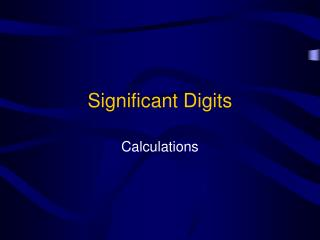DownloadDownload PresentationSignificant Digits

# Significant Digits

Download Presentation## Significant Digits

- - - - - - - - - - - - - - - - - - - - - - - - - - - E N D - - - - - - - - - - - - - - - - - - - - - - - - - - -
##### Presentation Transcript

1. Significant Digits Calculations

2. Significant Digits in Calculations • Now you know how to determine the number of significant digits in a number • How do you decide what to do when adding, subtracting, multiplying, or dividing?

3. Rules for Addition and Subtraction • When you add or subtract measurements, your answer must have the same number of decimal places as the one with the fewest • For example: 20.4 + 1.322 + 83 = 104.722

4. Addition & Subtraction Continued • Because you are adding, you need to look at the number of decimal places 20.4 + 1.322 + 83 = 104.722 (1) (3) (0) • Since you are adding, your answer must have the same number of decimal places as the one with the fewest • The fewest number of decimal places is 0 • Therefore, you answer must be rounded to have 0 decimal places • Your answer becomes • 105

5. 1.23056 + 67.809 = 23.67 – 500 = 40.08 + 32.064 = 22.9898 + 35.453 = 95.00 – 75.00 = 69.03956  69.040 - 476.33  -476 72.1440  72.14 58.4428  58.443 20  20.00 Addition & Subtraction Problems

6. Rules for Multiplication & Division • When you multiply and divide numbers you look at the TOTAL number of significant digits NOT just decimal places • For example: 67.50 x 2.54 = 171.45

7. Multiplication & Division • Because you are multiplying, you need to look at the total number of significant digits not just decimal places 67.50 x 2.54 = 171.45 (4) (3) • Since you are multiplying, your answer must have the same number of significant digits as the one with the fewest • The fewest number of significant digits is 3 • Therefore, you answer must be rounded to have 3 significant digits • Your answer becomes • 171

8. 890.15 x 12.3 = 88.132 / 22.500 = (48.12)(2.95) = 58.30 / 16.48 = 307.15 / 10.08 = 10948.845  1.09 x 104 3916.977  3917.0 141.954  142 3.5376  3.538 30.47123  30.47 Multiplication & Division Problems

9. 18.36 g / 14.20 cm3 105.40 °C –23.20 °C 324.5 mi / 5.5 hr 21.8 °C + 204.2 °C 460 m / 5 sec = 1.293 g/cm3 = 82.20 °C = 59 mi / hr = 226.0 °C = 90 or 9 x 101 m/sec More Significant Digit Problems